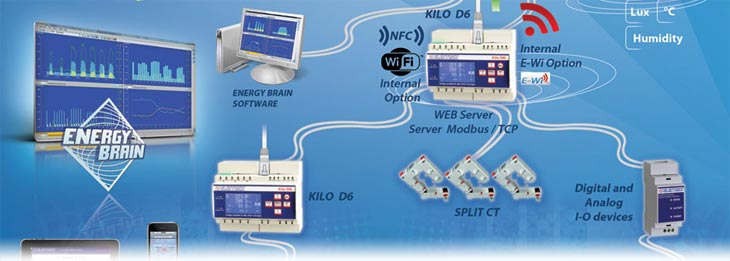An improper value of the power of the CT and / or an under-dimensioned connecting cable of the CT , may lead to distortions and significant attenuation of the signal and thus the measure can be incorrect.

For the measurement of the powers, in particular the active one, it is essential that the value of current and the phase angle of the secondary of the CT are correct.

– The power of the CT must be sized for the load on the secondary taking into consideration the section and the length of the cable.

– The size of the cable must consider the value of power delivered by the CT as a function of the circulating current, of the distance to be covered and of the load on the CT.

The Electrex instruments have the advantage of not involving practically no load on the secondary of the CT.
Therefore, what discussed above, leads to some practical simplifications.
We can therefore apply the following formula, an empirical one, but effective:
VA *  mm2 = A *  L
where
VA = Rated capacity of the CT in VA
mm2 = Section in mm2 of the cable used to connect the CT to the instrument
A = Maximum current output of the CT secondary in Amperes
L = Length of the cable (the distance between the CT and the instrument, expressed in meters)
NOTE: it is not needed to double the value because the formula already takes into account the outward and return of the cable.

So, for calculating the power of the CT:

VA = A *  L / mm2
while to calculate the cable section:
mm2 = A *  L / VA